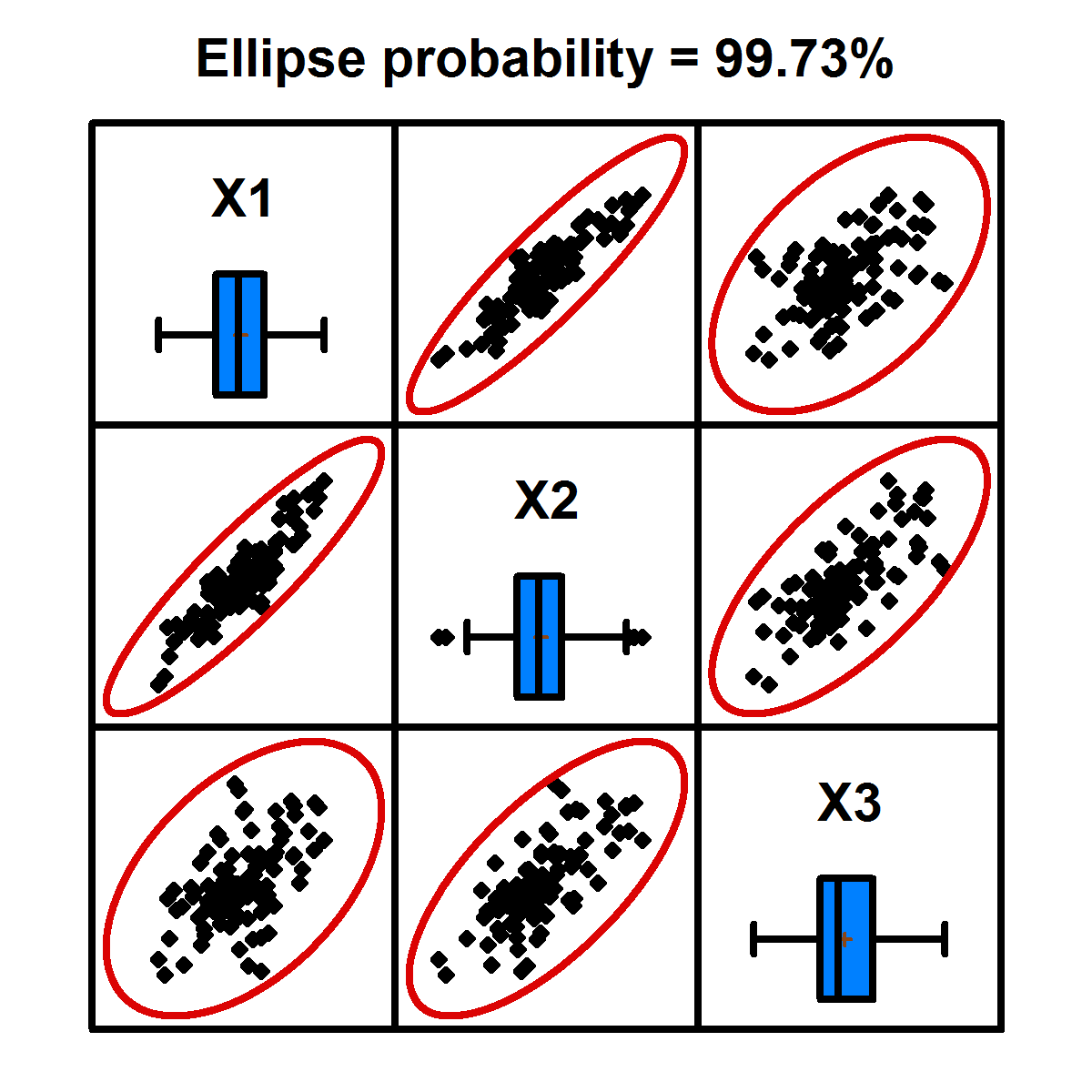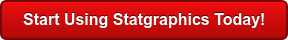# Monte Carlo Simulation

Monte Carlo simulation is used to estimate the distribution of variables when it is impossible or impractical to determine that distribution theoretically. It is used in many areas, including engineering, finance, and DFSS (Design for Six Sigma). A typical Monte Carlo simulation includes: (1) One or more input variables X, some of which usually follow a probability distribution. (2) One or more output variables Y, whose distribution is desired. (3) A mathematical model coupling the X’s and the Y’s. Statgraphics also includes a wide array of random number generators for use in simulation models.Procedure Statgraphics Centurion 18 Statgraphics
Sigma express
Statgraphics
stratus
Statgraphics
Web Services
StatBeans
General Simulation ModelsARIMA Time Series ModelsRandom Number GenerationMultivariate Normal Random Numbers#### General Simulation Models

This procedure is used to find the distribution of one or more output variables defined by mathematical models coupling them with one of more input variables. A probability distribution for each input variable may be specified. The primary output includes estimated percentiles for the output variables.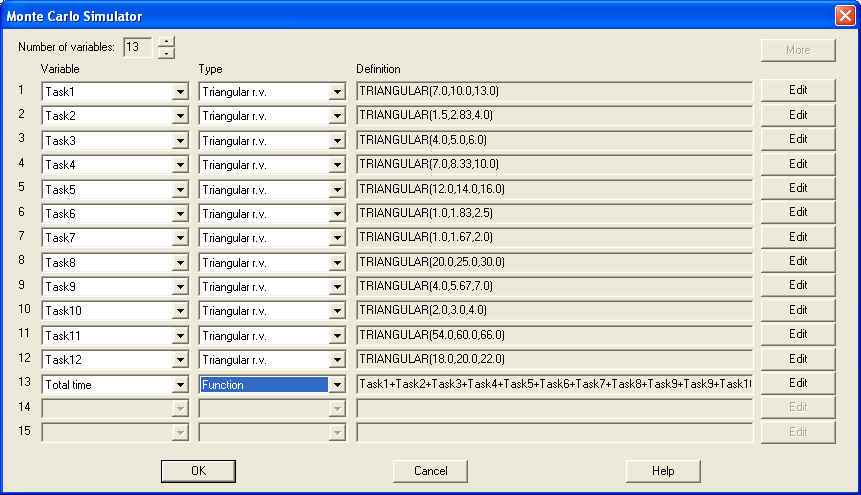#### ARIMA Time Series Models

This procedure generates random samples from ARIMA time series models.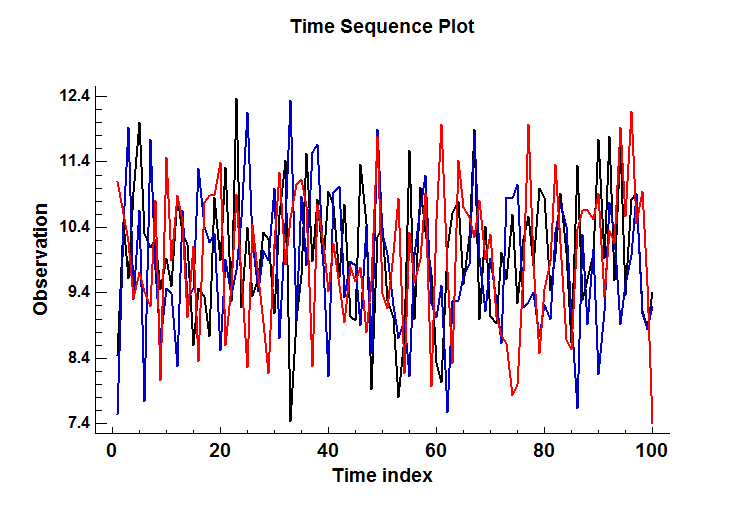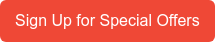#### Random Number Generation

It is frequently necessary to generate random numbers from different probability distributions. This procedure simplifies the process of creating multiple samples of random numbers. 49 probability distributions are available.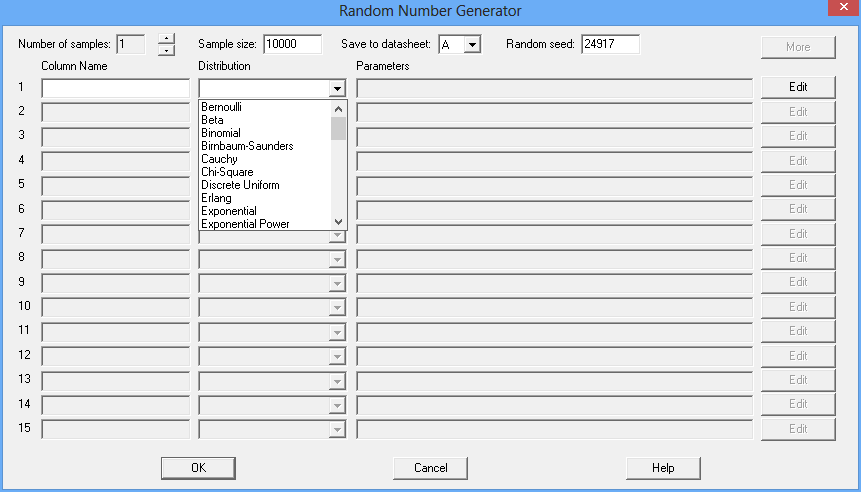#### Multivariate Normal Random Numbers

This procedure generates random numbers from a multivariate normal distribution involving up to 12 variables. The user inputs the variable means, standard deviations, and the correlation matrix. Random samples are generated which may be saved to the Statgraphics databook.